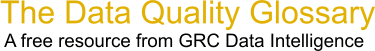Choose Index below for a list of all words and phrases defined in this glossary.

# Analysis of Variance (ANOVA)

index | Index

## Analysis of Variance (ANOVA) - definitions

Analysis of Variance (ANOVA) - A basic statistical technique for determining the proportion of influence a factor or set of factors has on total variation. It subdivides the total variation of a data set into meaningful component parts associated with specific sources of variation to test a hypothesis on the parameters of the model or to estimate variance components. There are three models: fixed, random and mixed.

[Category=Quality ]

Source: American Society for Quality, 31 August 2010 10:29:00, http://www.asq.org/glossary/These advertisers support this free service

Analysis of Variance (ANOVA) - Analysis of variance is a statistical technique for analyzing data that tests for a difference between two or more means by comparing the variances within groups and variances between groups. See the tool 1-Way ANOVA.

[Category=Data Quality ]

ANOVA - ANalysis Of VAriance (ANOVA), a calculation procedure to allocate the amount of variation in a process and determine if it is significant or is caused by random noise. A balanced ANOVA has equal numbers of measurements in each group/column. A stacked ANOVA: each factor has data in one column only and so does the response.

[Category=Data Quality ]

Source: iSixSigma, 30 December 2010 08:14:59, http://www.isixsigma.com/index.php?option=com_glossaryAnalysis of Variance (ANOVA) - a basic statistical technique for analyzing experimental data. It subdivides the total variation of a data set into meaningful component parts associated with specific sources of variation in order to test a hypothesis on the parameters of the model or to estimate variance components. ANOVA is used to test whether the means of many samples differ but it does so using variation instead of mean. It compares the amount of variation within the samples to the amount of variation between the means of samples. If the 'between variation' is significantly larger than the 'within variation', we conclude that the mean of our response has changed. An excellent tool to help visualize an ANOVA analysis is a multiple box plot - showing the mean (dot) and the within group variation (box). ANOVA provides an effective technique to separate inherent variance and special cause variance (i.e., group effect variation). ANOVA also provides a methodology to evaluate the robustness of a process to various levels of a particular factor (or treatment).

[Category=Quality ]

Source: The Quality Portal, 30 March 2011 07:57:19, http://thequalityportal.com/glossary/a.htmanalysis of variance - [statistics] A statistical procedure used to evaluate the variance of the mean values for two or more datasets in order to assess the probability that the data comes from the same sample or statistical population.

[Category=Geospatial ]

Source: esri, 16 January 2012 09:11:00, http://support.esri.com/en/knowledgebase/GISDictionary/term/abbreviationData Quality Glossary.  A free resource from GRC Data Intelligence. For comments, questions or feedback: dqglossary@grcdi.nl